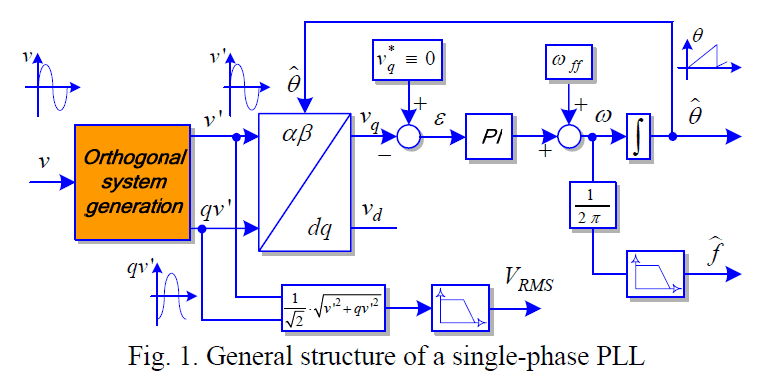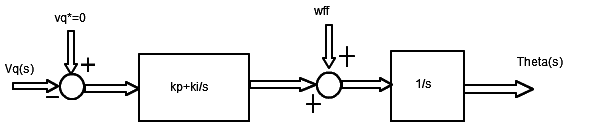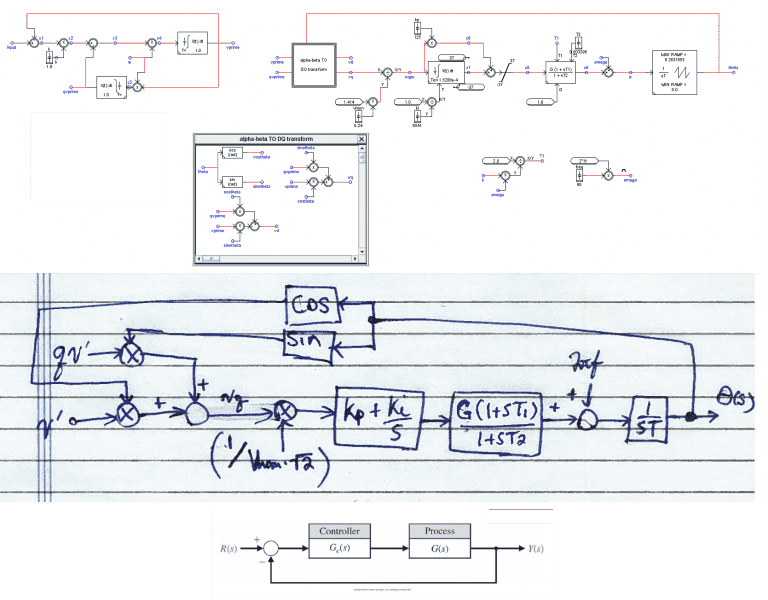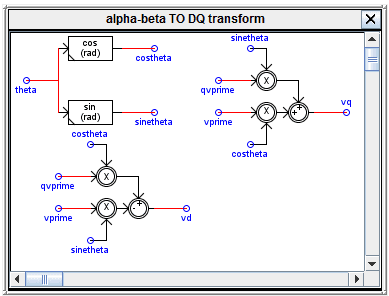Tuning PI Controller in PLL

Hello,

I've written a program that executes the following control diagram.Now I am trying to calculate the PI controller gains such that I can achieve a settling time of 0.06s and a damping factor of 1.

How can I do this?

I tried sketching a control diagram of the relevant portions.Thanks!

Attachments

Hesch
Gold Member

In the latter diagram you are missing the feed-back loop ( from Theta(s) to Vq(s) ).
Use Masons rule to find the closed-loop transfer-function ( and the characteristic equation ) of the system.
Create root-curves, varying kp and ki using some computerprogram (CAD), until the characteristic roots fits your specifications.

Advice: Use z-transform instead of Laplace-transform, as z-transform calculates correctly despite discreet and time-delayed calculations.

In the latter diagram you are missing the feed-back loop ( from Theta(s) to Vq(s) ).
Use Masons rule to find the closed-loop transfer-function ( and the characteristic equation ) of the system.
Create root-curves, varying kp and ki using some computerprogram (CAD), until the characteristic roots fits your specifications.

Advice: Use z-transform instead of Laplace-transform, as z-transform calculates correctly despite discreet and time-delayed calculations.

Hi,

Below I've included a more detail diagram of the controls, and another sketch of the control diagram.If I can convert this into something similar to the diagram shown at the bottom of the figure, then I can do some analysis such as root locus or by using MATLAB to make a sensible choice for kp and ki given the constraints. But how can I get it into this form?

EDIT: I worked on writing down the equations for this control diagram and got the following,

$$\frac{1}{T Vnom s^{2} \left(T_{2} s + 1\right)} \left(\frac{\sqrt{2} G}{2} \left(ki + kp s\right) \left(T_{1} s + 1\right) \left({qv}' \sin{\left (\theta(s)) \right )} + {v}' \cos{\left (\theta(s)) \right )}\right) - T Vnom s^{2} \theta(s)) \left(T_{2} s + 1\right) + Vnom \omega s \left(T_{2} s + 1\right)\right) = 0$$

but now I need to solve for the transfer function somehow.

Thanks!

Attachments

Last edited:
Hesch
Gold Member
I can do some analysis such as root locus or by using MATLAB to make a sensible choice for kp and ki given the constraints. But how can I get it into this form?

I don't quit understand what you mean by "get into this form", but anyway:

In your diagram, drawn by hand, I can count about 1 zero and 3 poles (3. order system), and as such you must have 3 tuning-possibilities. You can add an extra zero in your controller, thereby having a PID-controller to comply with your specifications.

Generally having a control-loop with forward feed = A(s) and backward feed = B(s), Masons rule says that the transferfunction of the whole system will be:

y(s)/u(s) = A(s) / ( 1 + A(s)*B(s) )

Setting the denominator = 0, you have the characteristic equation, giving the characteric roots at different values of kp, ki, kd. Now keep two of the values fixed while varying the third (iteratively). It's a "cut and try" proces, which ( after some training ) can be done in 5 minutes.

Note: If your tuned system is not physically doing exactly as intended, the cause may be that you are using Laplace-transform instead of z-transform!

Hello,

I've still been working on this problem for the past couple days and feel as though I've better expressed my concerns in the post below.

Let's start over.

Evening gents,

I wrote a program that executes the following control diagram. The contents of the orange box titled, "Orthogonal system generation" is shown in the lower part of the Figure as indicated by the black arrow.

I did some analysis by hand to better understand how this control diagram works and have attached my scribbles, but I still have some unanswered questions.

1. I've read in several other papers that the output of the PI controller is $$\Delta \omega$$. In my analysis I found that the input error signal to the PI controller was, $$\epsilon = V_{m} ( \phi - \hat{\phi} )$$, and I cannot figure out how this becomes $$\Delta \omega$$ at the output. Any ideas?
2. In the PI controller the output of the integrator in the integral path has a limiter at the output. What I'm confused about is how one should set these limits. Through experimentation with my program, I found that these limits will limit that amount of frequency deviation you allow in the output frequency signal $$\omega$$. This in turn affects the speed of the response for the controller when the phase of the input signal given undergoes a step change. The more frequency deviation you allow (i.e. the larger your limits are) the faster the response of the controller (i.e. it will lock onto the phase of the signal faster). However, as a trade off for faster controller response, one observes more deviation in the frequency signal $$\omega$$. Is there a way I can do some analysis to robustly determine how these limits affect the performance of the controller? Some guidance is all I'm asking for.

donpacino
Gold Member
what is in the block that ouputs Vq

Hesch
Gold Member
What kind of bandwidth do you need. how much jitter is acceptable

I've guessed that the application could be speed-control of a sensorless ac-motor.

Hi Don and Hesch!

It seems I didn't attached my hand written notes to my previous post, so I'll attach them now. Please see the attached pdf.

Also, the portion inside the block that outputs Vq is a Clarke to Park transform. It looks like this,I tried to write the program in a flexible way so that the user can vary different parameters based on their specific requirements. One example of this is allowing the user to enter a minimum, nominal and maximum frequency. This entered values would then be used to calculate the appropriate limits for the integral path in the PI controller.

One application could be for grid-connected inverter systems. These systems rely on accurate and fast detection of the phase angle, the amplitude and the frequency of utility voltage in order to guarantee the correct generation of the reference signals. (This is taken directly from the paper on which I based the program)

I'm still trying to figure out how the output of the PI controller becomes the change is frequency in rad/s, and any other insight on how to set the limits in the integral path.

Thanks!

Attachments

Hesch
Gold Member
One application could be for grid-connected inverter systems. These systems rely on accurate and fast detection of the phase angle, the amplitude and the frequency of utility voltage in order to guarantee the correct generation of the reference signals. (This is taken directly from the paper on which I based the program)

I'm still trying to figure out how the output of the PI controller becomes the change is frequency in rad/s, and any other insight on how to set the limits in the integral path.

An example for a setup for a synchrounous motor is given here:

The system is composed of two loops: a "d"-loop and a "q"-loop, controlling a "d"-current and a "q"-current. The "d"-current will yield no torque in the motor as the magnetic field in the rotor and stator will be in parallel ( therefore Isd is typical set to zero. The "q"-current will yield a torque as the magnetic fields will be perpendicular.
The third input (ω) must be synchronous with the motor-speed, otherwise the motor will "fall out". The motorspeed will increase if you set a high torque (high Iq).

I don't quite undrestand what you mean by "limits in the integral path". The integrator must be able to yield maximum voltage for the three phases of the motor. The purpose of the PI-filter is to reduce current current-error (and noise).

I will not recommend one controller for a grid of inverters/motors. It will never be stable/robust. To get one motor stable/robust, using Clark-Park is hard enough. The intension by Clark-Park is to spare a sensor (encoder). Using an encoder will make the system a hundred times more accurate/robust/stable.

Last edited:
donpacino
Gold Member
Hi Don and Hesch!

It seems I didn't attached my hand written notes to my previous post, so I'll attach them now. Please see the attached pdf.

Also, the portion inside the block that outputs Vq is a Clarke to Park transform. It looks like this,I tried to write the program in a flexible way so that the user can vary different parameters based on their specific requirements. One example of this is allowing the user to enter a minimum, nominal and maximum frequency. This entered values would then be used to calculate the appropriate limits for the integral path in the PI controller.

One application could be for grid-connected inverter systems. These systems rely on accurate and fast detection of the phase angle, the amplitude and the frequency of utility voltage in order to guarantee the correct generation of the reference signals. (This is taken directly from the paper on which I based the program)

I'm still trying to figure out how the output of the PI controller becomes the change is frequency in rad/s, and any other insight on how to set the limits in the integral path.

Thanks!
the units before and after a controller like that will be the same.

SO think about what Vq is. WHat does it represent. WHen will it be zero. when will it be at it's max?